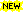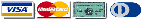InterReg 2014

Mathematical Software for Interpolation and Regression

30-Days Demo - for XP to Windows 10 (32 and 64-bit)

Interpolation and Regression are fundamental and important calculations in mathematics. Mr. Newton and Mr. Gauss were engaged in-depth with numerical solutions for these problems. Today, there are improved algorithms, that can solve such tasks.

InterReg allows you to do such complex calculations just with some point-and-click. So this program is not only for mathematics and engineers. The applications are many and reach from education over science to productive use in your company: in example a meaningful sales forecast can be done in minutes.

Over this, the software offers some other functionality to do curve sketching, integration and statistics as well.

InterReg Features

•Loading and saving of projects
•Undo/Redo
•Recalculate
• Calculates with arbitrary precision.
• Easy graphical usage and state-of-the-art algorithms
• Without a complicated scripting language you are able to do some complex calculations with simple point-and-click.
• So this software targets both mathematicians and non-mathematicians
• Modern algorithms give exact results
• Comprehensive import functions import sampling points from many different formats and databases
• Interpolation
• Linear Interpolation
• Cubic Splines
• Akima Splines
• Regression
• Regression of arbitrary functions using:
• LBFGS algorithm (quasi-Newton operation)
• Levenberg-Marquardt algorithm
• Curve Sketching
• Numerical derivations, zero crossings, extreme values, inflection points
• Crossings of two curves
• Tangents
• Integrals
• Integrals between the x-axis and between two curves:
• Romberg algorithm
• Simpson algorithm
• Statistics
• Arithmetic mean value
• Median
• Trend
• Correlation (Pearson and Spearman)
• Function Plotter
• Plotting of arbitrary function therms
• Other Features
• Cartesian coordinate system
• Optional date/time values on the abscissa
• Any number of graphs and value lists
• Printing
• Calculation and export of interpolated sample points

System Requirements

• All current versions of MS-Windows
• DotNet Framework 4.0+ (already installed on most computers)
~ 2.5 MB

Version 3.2.2 - english, german

Order

You can try out InterReg 2014 free of charge and without obligation for 30 days. After that you will need to obtain a user license if you wish to continue using this software.

The cost for a single user license** is € 24,90 net amount *

*) All prices are net amounts. If and how much VAT you have to pay depends on how and from where you place your order. More information can be found on the Shop Page

**) This license authorizes you to use the software on a single computer. There are no other costs. Free updates to all versions 2.x are included.

Order InterReg 2014Secure payment by credit card, bank transfer or PayPal
via the company Share-it Digital River GmbH, Koeln